# Table 2 Description of transfer functions for interrupted time series analysis in ARIMA

Function Values for h and  r Transfer function Response i at times 0 through k  post-intervention Form of response Interpretation
Step function
$${S}_t=\left\{\begin{array}{c}0, if\ t<T\\ {}1, if\ t\ge T\end{array}\right.$$
h = 0, r = 0 ω0 i0 = ω0
i1 = ω0
i2 = ω0

ik = ω0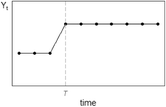The time series increases by ω0 immediately following the intervention, and remains at this new level for the duration of the study period.
h = 0, r = 1 $$\frac{\omega_0}{\left(1-{\delta}_1B\right)}$$
(|δ1| < 1)
i0 = ω0
i1 = ω0(1 + δ1)
$${i}_2={\omega}_0\left(1+{\delta}_1+{\delta}_1^2\right)$$

$${i}_k={\omega}_0\left(1+{\delta}_1+{\delta}_1^2+\dots +{\delta}_1^k\right)$$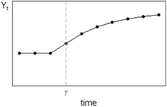The time series increases by ω0 immediately following the intervention, and increases by $${\omega}_0{\delta}_1^k$$ each subsequent time point until it reaches a new level, calculated by $$\frac{\omega_0}{\left(1-{\delta}_1\right)}$$.
Pulse function
$${P}_t=\left\{\begin{array}{c}0, if\ t\ne T\\ {}1, if\ t=T\end{array}\right.$$
h = 0, r = 0 ω0 i0 = ω0
i1 = 0
i2 = 0

ik = 0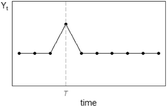The time series increases by ω0 immediately following the intervention and returns to baseline immediately afterwards.
h = 0, r = 1 $$\frac{\omega_0}{\left(1-{\delta}_1B\right)}$$
(|δ1| < 1)
i0 = ω0
i1 = ω0δ1
$${i}_2={\omega}_0{\delta}_1^2$$

$${i}_k={\omega}_0{\delta}_1^k$$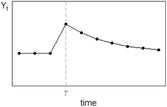The time series increases by ω0  the time of the intervention, and decays by (1 − δ1) each subsequent time point.
Ramp function
$${R}_t=\left\{\begin{array}{c}0, if\ t<T\\ {}t-T+1, if\ t\ge T\end{array}\right.$$
h = 0, r = 0 ω0 i0 = ω0
i1 = 2ω0
i2 = 3ω0

ik = (k + 1)ω0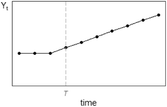The time series increases by ω0 at each time point.